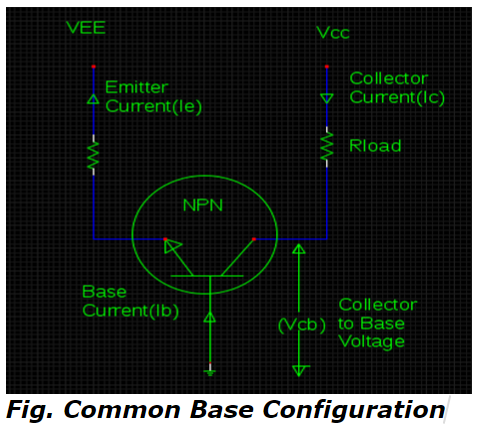# Significance of VCE, Beta Rule & Operating Regions - MCQs with answers

Q1. What value of load resistance (RL) generates collector to base voltage (VCB) of about 6V for the following circuit configuration ?a. 14 k Ω
b. 12 k Ω
c. 10 k Ω
d. 8 k Ω

Q2. Which rule clarifies an ideology of referring the resistance from one part of transistor to another of its parts similar to the strategy of using primary & secondary winding impedances of a transformer?

a. Alpha rule
b. Beta rule
c. Gamma rule
d.None of the above

Q3. It is possible to determine the operating state of transistor with an assistance of VCE under the category of well into saturation region if value of VCE is______.

a. only greater than zero
b. only equal to zero
c. only less than zero
d. greater than or equal to zero

ANSWER: c. only less than zero

Q4. The saturation condition of transistor implies that _______.

a. Collector current has highest possible value
b. entire Vcc gets dropped across load resistor
c. it acts as a closed switch with negligible value of resistance
d. all of the above